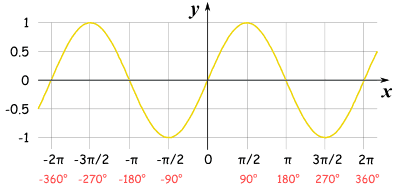# Sketch the graph of y= sin theta  for -pi <= theta <=pi?

Feb 20, 2018

See below.

#### Explanation:

To sketch this we must know 4 common points of the graph.

We know that $\pi$ in radians is ${180}^{\circ}$

This means that $\frac{\pi}{2}$ is ${90}^{\circ}$

From our knowledge, a sine graph has the coordinates:

$\left(0 , 0\right) \left(90 , 1\right) \left(180 , 0\right) \left(- 90 , - 1\right) \left(- 180 , 0\right)$

Converting these to radians we get:

$\left(0 , 0\right) \left(\frac{\pi}{2} , 1\right) \left(\pi , 0\right) \left(- \frac{\pi}{2}\right) \left(- \pi , 0\right)$

We know that a sine graph oscillates between $1$ and $- 1$.

This means we need to label the $y$ axis with the $1$ and $- 1$.

Along the $x$ axis we must go up in radians, use the points mentioned above.

Plot the points on the graph and sketch with a nice smooth curve.Notice that the graph repeats this pattern throughout.